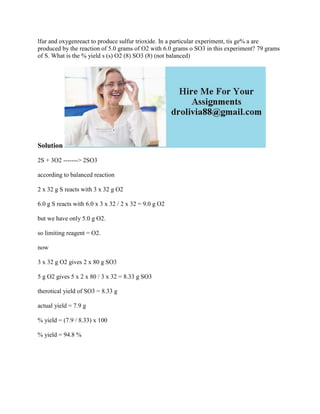Seu SlideShare está sendo baixado. ×

# lfur and oxygenreact to produce sulfur trioxide- In a particular exper.docx

Anúncio
Anúncio
Anúncio
Anúncio
Anúncio
Anúncio
Anúncio
Anúncio
Anúncio
Anúncio
AnúncioCarregando em…3
×

1 de 2 Anúncio

# lfur and oxygenreact to produce sulfur trioxide- In a particular exper.docx

lfur and oxygenreact to produce sulfur trioxide. In a particular experiment, tis ge% a are produced by the reaction of 5.0 grams of O2 with 6.0 grams o SO3 in this experiment? 79 grams of S. What is the % yield s (s) O2 (8) SO3 (8) (not balanced)
Solution
2S + 3O2 -------> 2SO3
according to balanced reaction
2 x 32 g S reacts with 3 x 32 g O2
6.0 g S reacts with 6.0 x 3 x 32 / 2 x 32 = 9.0 g O2
but we have only 5.0 g O2.
so limiting reagent = O2.
now
3 x 32 g O2 gives 2 x 80 g SO3
5 g O2 gives 5 x 2 x 80 / 3 x 32 = 8.33 g SO3
therotical yield of SO3 = 8.33 g
actual yield = 7.9 g
% yield = (7.9 / 8.33) x 100
% yield = 94.8 %
.

lfur and oxygenreact to produce sulfur trioxide. In a particular experiment, tis ge% a are produced by the reaction of 5.0 grams of O2 with 6.0 grams o SO3 in this experiment? 79 grams of S. What is the % yield s (s) O2 (8) SO3 (8) (not balanced)
Solution
2S + 3O2 -------> 2SO3
according to balanced reaction
2 x 32 g S reacts with 3 x 32 g O2
6.0 g S reacts with 6.0 x 3 x 32 / 2 x 32 = 9.0 g O2
but we have only 5.0 g O2.
so limiting reagent = O2.
now
3 x 32 g O2 gives 2 x 80 g SO3
5 g O2 gives 5 x 2 x 80 / 3 x 32 = 8.33 g SO3
therotical yield of SO3 = 8.33 g
actual yield = 7.9 g
% yield = (7.9 / 8.33) x 100
% yield = 94.8 %
.

Anúncio
Anúncio

### lfur and oxygenreact to produce sulfur trioxide- In a particular exper.docx

1. 1. lfur and oxygenreact to produce sulfur trioxide. In a particular experiment, tis ge% a are produced by the reaction of 5.0 grams of O2 with 6.0 grams o SO3 in this experiment? 79 grams of S. What is the % yield s (s) O2 (8) SO3 (8) (not balanced) Solution 2S + 3O2 -------> 2SO3 according to balanced reaction 2 x 32 g S reacts with 3 x 32 g O2 6.0 g S reacts with 6.0 x 3 x 32 / 2 x 32 = 9.0 g O2 but we have only 5.0 g O2. so limiting reagent = O2. now 3 x 32 g O2 gives 2 x 80 g SO3 5 g O2 gives 5 x 2 x 80 / 3 x 32 = 8.33 g SO3 therotical yield of SO3 = 8.33 g actual yield = 7.9 g % yield = (7.9 / 8.33) x 100 % yield = 94.8 %